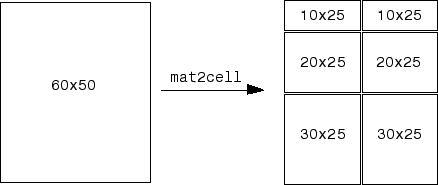# mat2cell

## 语法

``C = mat2cell(A,dim1Dist,...,dimNDist)``
``C = mat2cell(A,rowDist)``

## 说明

``C = mat2cell(A,dim1Dist,...,dimNDist)` 将数组 `A` 划分为更小的数组，并在元胞数组 `C` 中返回它们。向量 `dim1Dist,...dimNDist` 指定如何划分 `A` 的行、列和（如果适用）更高维度。`C` 中较小的数组可以具有不同大小。`A` 可以包含任何数据类型。`

``C = mat2cell(A,rowDist)` 将数组 `A` 划分为一个 `n`×1 元胞数组 `C`，其中 `n` 等于 `rowDist` 中元素的数量。`

## 示例

`A = reshape(1:20,5,4)'`
```A = 4×5 1 2 3 4 5 6 7 8 9 10 11 12 13 14 15 16 17 18 19 20 ```

`A` 划分为两个 2×3 和 2 个 2×2 子数组。以元胞数组形式返回子数组。

`C = mat2cell(A,[2 2],[3 2])`
```C=2×2 cell array {2x3 double} {2x2 double} {2x3 double} {2x2 double} ```

`celldisp(C)`
``` C{1,1} = 1 2 3 6 7 8 C{2,1} = 11 12 13 16 17 18 C{1,2} = 4 5 9 10 C{2,2} = 14 15 19 20 ```

`A = reshape(1:20,5,4)'`
```A = 4×5 1 2 3 4 5 6 7 8 9 10 11 12 13 14 15 16 17 18 19 20 ```

```rowDist = [1 3]; C = mat2cell(A,rowDist)```
```C=2×1 cell array {[1 2 3 4 5]} {3x5 double } ```

`celldisp(C)`
``` C{1} = 1 2 3 4 5 C{2} = 6 7 8 9 10 11 12 13 14 15 16 17 18 19 20 ```

## 输入参数

```C = mat2cell(A,[10 20 30],[25 25]) ``````A = rand(3,0,4); C = mat2cell(A,[1 2],[],[2 1 1]); ```

`rowDist` 的每个元素指定 `C` 中对应元胞所含子数组的行数。`rowDist` 的元素总和必须等于 `A` 的行数。